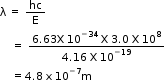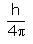Courses

# Test: Dual Nature Of Matter

## 10 Questions MCQ Test Chemistry Class 11 | Test: Dual Nature Of Matter

Description
This mock test of Test: Dual Nature Of Matter for Class 11 helps you for every Class 11 entrance exam. This contains 10 Multiple Choice Questions for Class 11 Test: Dual Nature Of Matter (mcq) to study with solutions a complete question bank. The solved questions answers in this Test: Dual Nature Of Matter quiz give you a good mix of easy questions and tough questions. Class 11 students definitely take this Test: Dual Nature Of Matter exercise for a better result in the exam. You can find other Test: Dual Nature Of Matter extra questions, long questions & short questions for Class 11 on EduRev as well by searching above.
QUESTION: 1

### A photon has energy of 2.6 eV. What is its wavelength?

Solution:

Given, 2.6 e V = 2.6 X 1.6 X 10-19 J = 4.16 X 10-19 J

Now,by using formula,QUESTION: 2

### If uncertainty in the position of an electron is zero, the uncertainity in its momentum would be

Solution:

Actually, according to uncertainty principle,

Δx.Δp ≥ h/4π

or Δx.Δp ≈ h/4n

where, Δx, is uncertainty in position and Δp is uncertainty in momentum.

So, if Δx = 0

Hence, uncertainty in momentum Δp = h/4π x 0 = Infinity.

QUESTION: 3

### Uncertainty in the position of an electron (mass 9.1 x 10-31 kg) moving with a velocity of 300 ms-1, accurate upto 0.001% will be:

Solution:

Change in position (X),
P= momentum,
P=mv.
X ×P = h/4π.
X× m v = h/4π.
X= h/4πmv.
X=6.625×10-34/4 ×3.14×9.1×10^-31×300.
X=6.625×10-5/342.8.
X=0.0192×10^-5.
X= 19.2× 10-7.
in question it is given to take accurate up to 0.001%.= 1×10-3/100=1 ×10-5.
X= 19.2 ×10-7/10-5.
X=19.2 × 10-2.

QUESTION: 4

What experimental result did Albert Einstein cite as evidence that light has particle-like properties?

Solution:

Einstein's explanation of photoelectric effect using the concept of photons. In 1905, Albert Einstein provided an explanation of the photoelectric effect, an experiment that the wave theory of light failed to explain. He did so by postulating the existence of photons, quanta of light energy with particulate qualities.

QUESTION: 5

The de Broglie wavelength of a particle is given by.

Solution:

According to De Broglie, a moving small particle like electron, proton, neutron, dust particle, a small ball etc. has the properties of a wave (Dual nature of matter).

The wave length of a moving particle may be calculated as

λ=h/mv =h/p

De Broglie equation

λ =wave length

h= planck’s constt.

m=mass of particle

v=velocity of particle

p=momentum of the particle

QUESTION: 6

The de – Broglie wavelength of an electron is 600 nm. The velocity of the electron having the mass 9.1 X 10-31 Kg is

Solution:

1nm= 10^-9m.
wavelength= 600nm= 600 ×10-9m
wavelength= h/p.
wavelength= h/mv
v= h/ m wavelength
v=6.625×10^-34/9.1×10^-31×600× 10-9.
v= 6.625 × 10+4/9.1 ×6.
v= 6.625× 10+4/9.1×6.
v= 6.625 ×104/54.6.
v= 0.12 × 104.
v= 0.0012 × 10+6 .

QUESTION: 7

In the relationship ∆x. ∆p =, ∆p is:

Solution:

The uncertainty principle is alternatively expressed in terms of a particle’s momentum and position. The momentum of a particle is equal to the product of its mass times its velocity. Thus, the product of the uncertainties in the momentum and the position of a particle equals h/(4π)

QUESTION: 8

The uncertainty principle states that

Solution:

The Heisenberg uncertainty principle states that it is impossible to know simultaneously the exact position and momentum of a particle. That is, the more exactly the position is determined, the less known the momentum, and vice versa.

QUESTION: 9

Whch of the following will have the maximum value of ∆v . ∆x

Solution:

This is accordance with the Heisenberg's uncertainty principle.It states that it is not possible to accurately determine the position and momentum of a microscopic particle such as electron simultaneously.

QUESTION: 10

The de Broglie wavelength of an electron is 8.7 x 10-11 m. The mass of an electron is 9.1 x 10-31 kg. The velocity of this electron is:

Solution:

Wavelength = h/p = h/ mv.
v= h/ m.wavelength
v= 6.625 × 10-34/9.1×10-31 × 8.7 × 10-11
v= 6.625 × 108/ 9.1× 8.7
v= 0.08367 ×108.